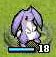# 星之一角

What have you found for these years?

## 2012-04-05

### thread-safe is not fiber-safe; fiber-safe could be even harder (3)

Other posts:
2148. 04-04 thread-safe is not fiber-safe; fiber-safe could be even harder
2149. 04-04 thread-safe is not fiber-safe; fiber-safe could be even harder (2)
2151. 04-05 thread-safe is not fiber-safe; fiber-safe could be even harder (3)

So I have done the Haskell equivalent for last examples.
To run these examples, you need to `cabal install monad-coroutine`
P.S. You can find all the codes in my sandbox/haskell/coroutine

## deterministic race conditions in coroutines

``````import Data.IORef (IORef, newIORef, readIORef, writeIORef)

type Fiber = Coroutine (Yield ()) IO ()

fiber :: IORef Int -> Fiber
fiber i = do
yield ()
lift (writeIORef i (t + 1))
return ()

main = do
i <- newIORef 0
let a = fiber i
b = fiber i in do
readIORef i >>= print -- 0
Left (Yield _ a') <- resume a
Left (Yield _ b') <- resume b
resume a'
resume b'
readIORef i >>= print -- should be 2``````

## STM cannot help here

``````import Control.Concurrent.STM (atomically, retry, TVar, STM,

type Fiber = Coroutine (Yield ()) STM ()

fiber :: TVar Int -> Fiber
fiber i = do
yield ()
lift (writeTVar i (t + 1))
return ()

main = do
i <- atomically (newTVar 0)
let a = fiber i
b = fiber i in do
atomically (readTVar i) >>= print -- 0
Left (Yield _ a') <- atomically (resume a)
Left (Yield _ b') <- atomically (resume b)
atomically (resume a')
atomically (resume b')
atomically (readTVar i) >>= print -- should be 2``````

## mutex, i.e. MVar is a better solution here, but beware of deadlock!

``````import Control.Concurrent.MVar (MVar, newMVar, readMVar, takeMVar, putMVar)

type Fiber = Coroutine (Yield ()) IO ()

fiber :: MVar Int -> Fiber
fiber i = do
t <- lift (takeMVar i)
yield ()
lift (putMVar i (t + 1))
return ()

main = do
i <- newMVar 0
let a = fiber i
b = fiber i in do
readMVar i >>= print -- 0
Left (Yield _ a') <- resume a
Left (Yield _ b') <- resume b -- deadlock
return ()``````

## The real solution with tryTakeMVar

``````import Control.Concurrent.MVar (MVar, newMVar, readMVar, tryTakeMVar, putMVar)

type Fiber = Coroutine (Yield ()) IO ()

fiber :: MVar Int -> Fiber
fiber i = lift (tryTakeMVar i) >>= \t ->
case t of
Nothing -> yield () >> fiber i
Just t  -> do
yield ()
lift (putMVar i (t + 1))
return ()

schedule :: [Fiber] -> IO ()
schedule []     = return ()
schedule (f:fs) = resume f >>= \t -> case t of
Left (Yield _ f') -> schedule (fs ++ [f'])
Right _           -> schedule  fs

main = do
i <- newMVar 0
let a = fiber i
b = fiber i in do
readMVar i >>= print -- 0
schedule [a, b]
readMVar i >>= print -- 2``````

## Conclusion

So after all this, my current conclusion is that, as every one might
already know, there's no silver bullet at all. We need threads, we
needs fibers (coroutines), we also need mutex (either with explicit
locking or not), and we also need STM in some cases. Use the right
tool for the right thing, after all... and never throw our old friends
(e.g. lock) away whether they are good or bad...

Long lived tools must have its values which cannot be easily replaced :o

#### 1 retries:

ngocnhung said...
This comment has been removed by a blog administrator.